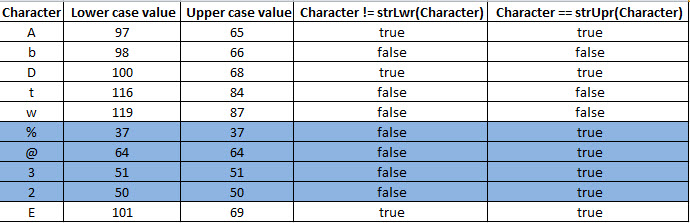Finance and operations

# Programming puzzle in X++: Is this character upper case?

I would like to share a logic puzzle that baffled me today (for some background, read this stack overflow question ). This will likely work in many programming languages, but obviously I am going to show you X++ code.

Problem:
Given the following string, write a job that displays a list of all the characters that are uppercase: AbDtw%@32E

SPOILER ALERT: I discuss my faulty solution as well as a better solution in the following section. If you want to try it first, stop reading here!

My faulty reasoning:

I assumed that you would write a loop that compared each character with it’s uppercase character and if the character is equal to it’s uppercase counterpart, the character must be uppercase. Let’s try that:

```static void findCapitalLetters(Args _args)
{
str testStr = "AbDtw%@32E";
int i;
int stringLenght = strLen(testStr);
str character;

for (i=1; i<=stringLenght; i+=1)
{
character = subStr(testStr, i, 1);
//If the character is EQUAL to it's uppercase counterpart, it must be uppercase:
if (char2num(testStr, i) == char2num(strUpr(testStr), i))
{
info(strFmt("'%1' at position %2 is an uppercase letter.", character, i));
}
}
}
```

OOPS! The characters %, @, 3 and 2 are evaluated as uppercase; this is not what I had in mind. So I assumed (wrongly again) that the answer would be to first check if the character is a letter (if it is a number or symbol, I can just ignore it). I was thinking along the lines of adding str2IntOk()…

The simple solution:
It turns out, if you reverse the question and ask, “Is this character not equal to its lower case letter?”, you get the correct answer:

```static void findCapitalLetters(Args _args)
{
str testStr = "AbDtw%@32E";
int i;
int stringLenght = strLen(testStr);
str character;

for (i=1; i<=stringLenght; i+=1)
{
character = subStr(testStr, i, 1);
//If the character is NOT EQUAL to it's lowercase counterpart, it must be uppercase:
if (char2num(testStr, i) != char2num(strLwr(testStr), i))
{
info(strFmt("'%1' at position %2 is an uppercase letter.", character, i));
}
}
}
```

See how subtle the difference it?

Explanation:
At first the two jobs looked the same to me. I thought the issue was with the way strLwr() and strUpr() work. It wasn’t until I examined the return values that I realized the problem is with the logical operators and not the string funtions.

Consider this table:If the character is a letter, it has a different value for upper and lower case. If the value is a symbol or number, it doesn’t have an upper or lower case, and the same value returned as upper and lower case.

In the case of numbers and symbols, the question “Is this character not equal to its lower case letter?” has a different answer than “Is this character equal to its upper case letter?”.

Of course, in both cases the real question you should ask yourself is: “What is the problem I am trying to solve?”.

Let me know in the comments if its only me, or if this baffled you for a moment too. Happy problem solving!